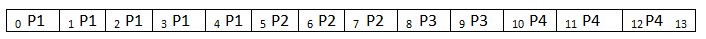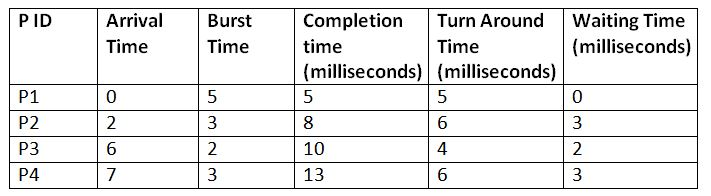# FCFS: First Come First Serve Scheduling Algorithm

Submitted by Monika Sharma, on June 22, 2019

The FCFS, which stands for First Come First Serve Scheduling Algorithm, is a non-preemptive scheduling algorithm, which means that if a process once starts executing in the processor, then it cannot be preempted in between the processing. Thus, the concept of priority and urgency is not implemented by this type of algorithm. In the FCFS algorithm, the process gets executed in the same sequence in which they enter the Ready state. It simply follows the strategy of "First come First serve with special services provided to none."

Now let us try to understand this further with the help of an example. Suppose there are four processes with process ID's P1, P2, P3, and P4 and they enter into the CPU as follows:

Process ID Arrival Time
(milliseconds)
Burst Time
(milliseconds)
P105
P223
P362
P473

So, if the OS follows the FCFS algorithm for scheduling these processes, then they will be executed in the following manner:

Gant Chart:```    Total Turn around Time = 5 + 6 + 4 + 6
= 21 milliseconds
Average Turn Around Time= Total Turn Around Time / Total No. of Processes
= 21 / 4
= 5.25 milliseconds

Total Waiting Time = 0 + 3 + 2 + 3
= 8 milliseconds
Average Waiting Time = Total Waiting Time / Total No. of Processes
= 8 / 4
= 2 milliseconds
```

What's New

Top Interview Coding Problems/Challenges!

Languages: » C » C++ » C++ STL » Java » Data Structure » C#.Net » Android » Kotlin » SQL
Web Technologies: » PHP » Python » JavaScript » CSS » Ajax » Node.js » Web programming/HTML
Solved programs: » C » C++ » DS » Java » C#
Aptitude que. & ans.: » C » C++ » Java » DBMS
Interview que. & ans.: » C » Embedded C » Java » SEO » HR
CS Subjects: » CS Basics » O.S. » Networks » DBMS » Embedded Systems » Cloud Computing
» Machine learning » CS Organizations » Linux » DOS
More: » Articles » Puzzles » News/Updates